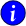# AIOMFAC-web Input Form

To run the model using input files from previous sessions click here.

JavaScript must be enabled in your browser to use this form. Please change your browser settings to proceed.

### WaterWater

Indicate whether water is a component of the liquid mixture or not.
Water will be system component no. 1 when set as present.

Yes, water is present in the system.
No, water is not present in the system.

### Organic ComponentsOrganic Components

Define an organic system component either

1. by selecting it from a predefined list of common organic compounds or

2. by defining the AIOMFAC subgroups and corresponding numbers of subgroup units of a component.

Learn about the nomenclature and correct use of subgroups.

+ Add Organic Component from     or

### Inorganic IonsInorganic Components
• Select a cation and a corresponding anion to define an electrolyte unit.

• Each electrolyte (salt/acid) component must have overall neutral charge, therefore the number of cations and anions in a unit is automatically set.

• Enter electrolytes consisting of more than one different anion and/or cation as split into different inorganic cation-anion pairs (i.e., as different components).

+

### Mixture Composition ScaleComposition Scale of Data Points
• Mass fraction definition: wi = niMij(njMj); with nj denoting moles of mixture components and Mj the corresponding molar masses.

• Input composition in mole fractions is here defined as mole fractions with respect to undissociated electrolyte components (i.e., salt/acid units and not dissociated ions).

• Mole fraction definition: x'i = nij(nj); with nj denoting moles of the mixture components.

• Note: in the presence of electrolyte components, this is a different mole fraction scale than the one used in the AIOMFAC output tables (hence x'i); in the output tables on the results page, the mole fractions (xi) are calculated on the basis of completely dissociated ions.

Mass Fractions
Mole Fractions

### Temperature and Composition

(1 to 101 data points)

T [K], mass or mole fractions of components:Setting Temperature and Composition of Data Points

An example of correct input of data points is shown on the Hints & Examples page.

• The first column is to set the temperature pertaining to a data point. (Click here to learn about the applicable temperature range.)

• Followed by the mass or mole fraction value of the second component. The mass or mole fraction value of component no. 1 will be set automatically by AIOMFAC (the sum of all mass or mole fractions has to be equal to 1.0). If water is present in the system, it will be component no. 1.

• The mole/mass fraction values of the remaining components follow in the order listed on the input-field header.

• Table columns must be separated by a "space", "tab", or "comma + space".

• Period (.) should be used as decimal point.

• The web model allows input of up to 101 data points per model run.

Show results in a new browser tab

© 2012–2023 Andreas Zuend, California Institute of Technology | McGill University last page update: 2021-12-09